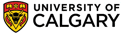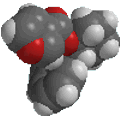Chapter 3: Conformations of Alkanes and Cycloalkanes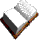Cycloalkanes

• Cycloalkanes literally means "cyclic alkanes", which means ring structures containing only C-C and C-H bonds.
• The C atoms in cycloalkanes are predicted to be sp3 hybridised which requires optimal C-C-C bond angles of 109.5o
• These structural units are commonly encountered in natural compounds such as steroids, with cyclopentanes and cyclohexanes being the most common.
• Other than the smallest ring system cyclopropane (which must be planar), cycloalkanes are "puckered"
• Puckering typically reduces ring strain (i.e. makes it more stable) by lowering torsional strains but this is offset by slightly increased angle strain.
• Ring strain : cyclopropane > cyclobutane > cyclopentane > cyclohexane

The most stable conformations of the first three cycloalkanes (the smallest three) are shown below where they can be contrasted with the planar conformation. In order to be able to compare the strain in each member of the cycloalkane series, the heat of combustion per methylene (i.e. -CH2-) is also given (see note below the table). The smaller this number is the less ring strain there is.
In each case, you should manipulate the 3D-JSMOL images to visualise the deviation from planarity and the effect this has on the eclipsing interactions of adjacent C-H bonds and C-C bonds (hint turn the model to "see" the Newman projections).

 planar most stable structure C3H6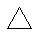CYCLOPROPANE ΔHc / CH2 = -697 kJ/mol (-166.6 kcal/mol) see left Analysis C4H8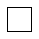CYCLOBUTANE ΔHc / CH2 = -681 kJ/mol (-162.7 kcal/mol) Analysis C5H10CYCLOPENTANE ΔHc / CH2 = -658 kJ/mol (-157.3 kcal/mol) Analysis Note: since the structures are not isomeric, we can't just compare the heats of combustion. However, simple cycloalkanes have have the generic molecular formula (CH2)n, where n = the number of C atoms in the ring. Therefore dividing the heat of combustion for each cycloalkane by n means we are able to make a reasonable comparison.© Dr. Ian Hunt, Department of Chemistry